## 1、什么是lstm

LSTM：长短期记忆网络（LSTM，Long Short-Term Memory）是一种时间循环神经网络，是为了解决一般的RNN（循环神经网络）存在的长期依赖问题而专门设计出来的，所有的RNN都具有一种重复神经网络模块的链式形式。

RNN 传送门：评论继续送书，史上最容易懂的RNN文章，小白也能看得懂

RNN的问题：存在梯度爆炸和消失的问题，对于长距离的句子的学习效果不好。

## 2、lstm 的网络结构

### 1、架构图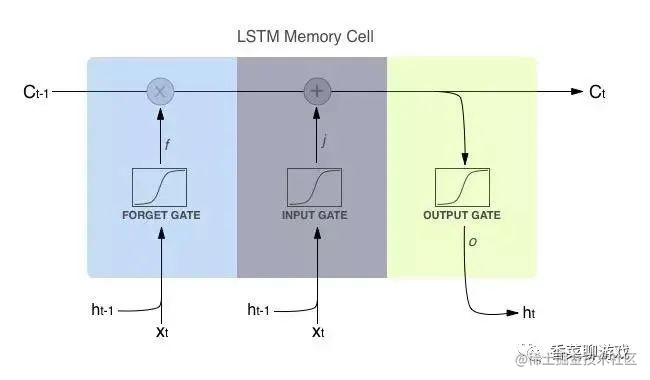x 也就是操作矩阵中对应的元素相乘，因此要求两个相乘矩阵是同型的

+号 则代表进行矩阵加法。

Ct-1 是 当前神经元的输出

Ht-1 是 隐藏层的参数

# 3、lstm 的门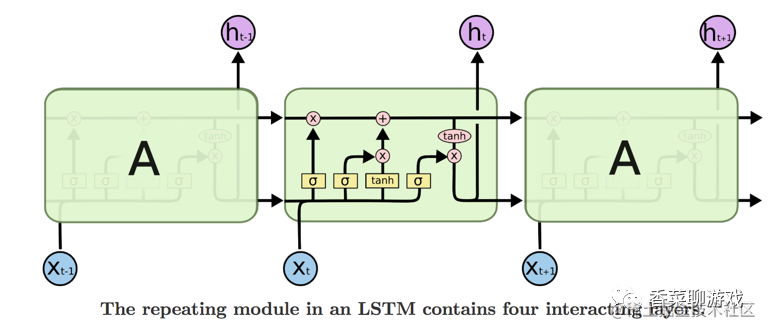### 3.2 lstm 的核心就是三个门函数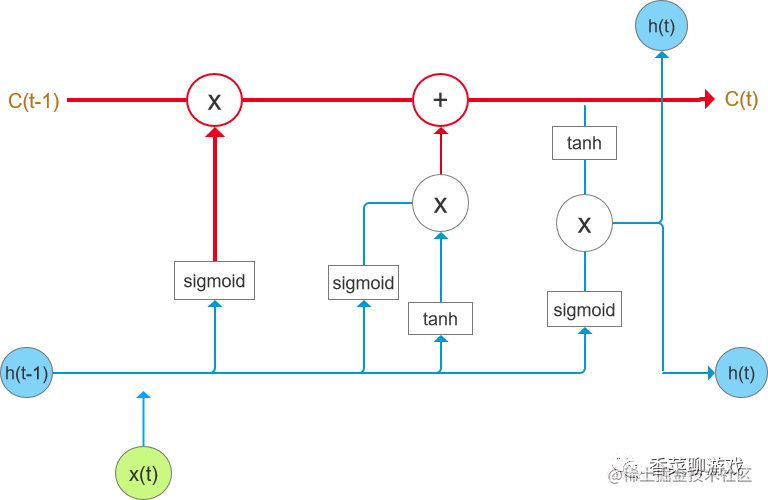tanh神经网络层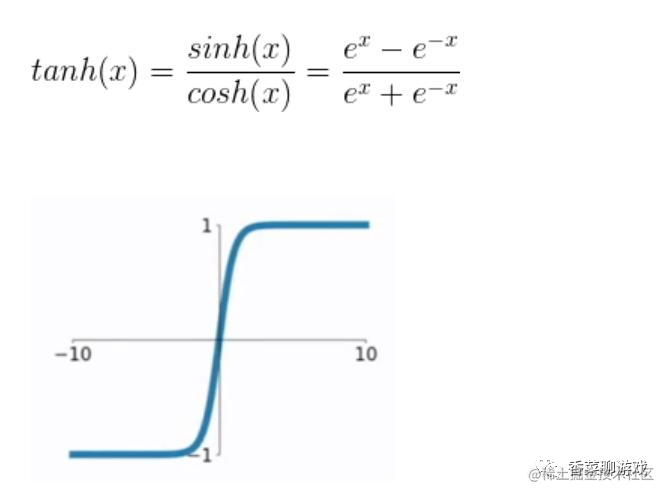sigmod神经网络层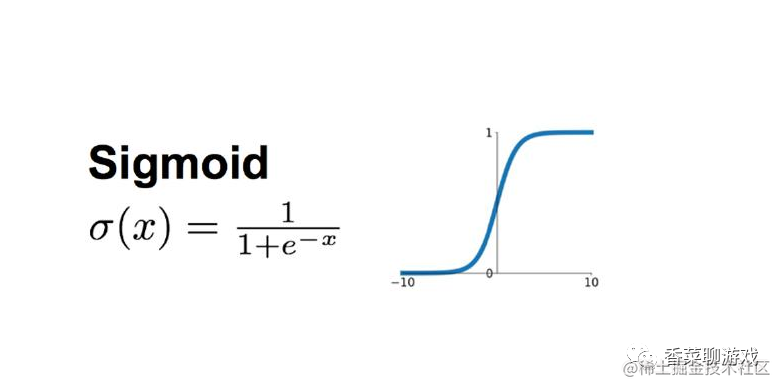### 3.3 遗忘门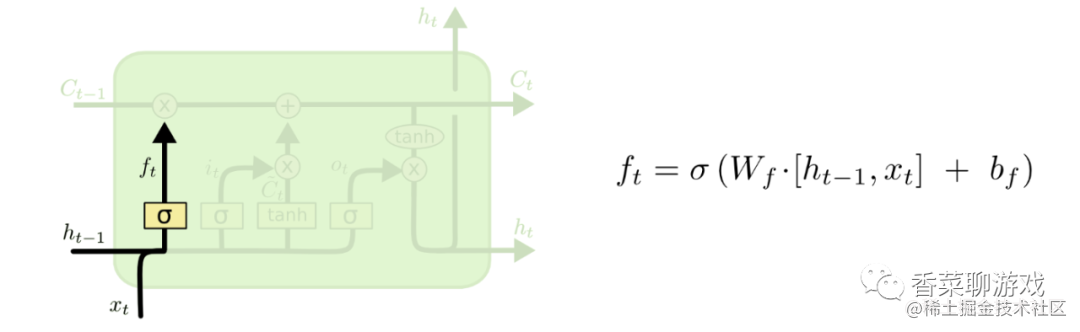Ht-1 = [0.1,0.2,0.3] 是一个一行三列的一个矩阵

Xt = [0.6,0.7,0.8] 也是一个一行三列的一个矩阵

6 代表一个神经单元，整个函数的模型就是 f = wx +b

Wf 是当前神经单元的参数

bf 是偏置参数

### 3.4 输入门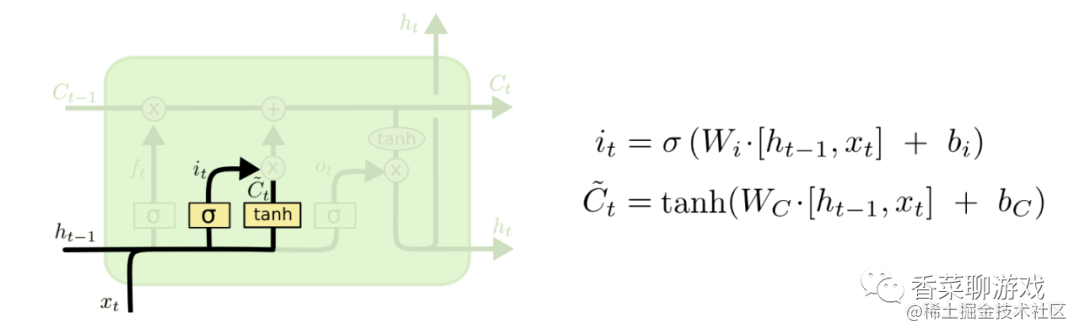It 的函数操作何上面的输入门的解释差不多，用以控制新状态信息的更新程度

Ct 用以控制输入的数据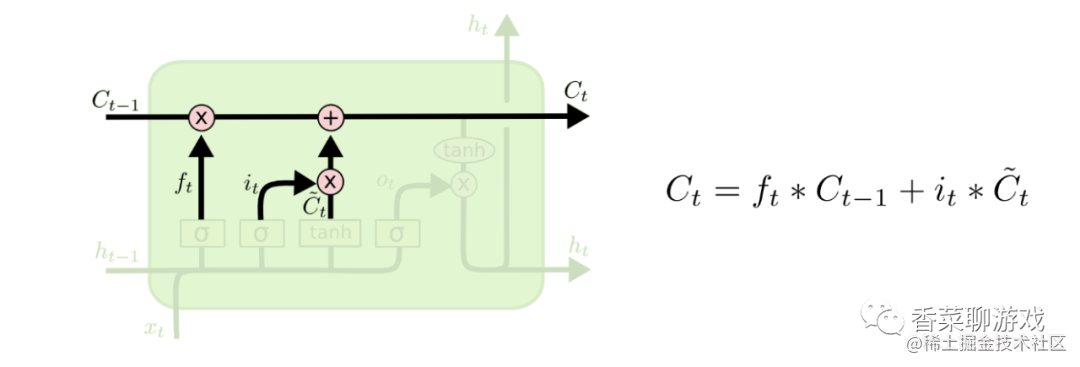Ct 就是当前时刻的输出

Ct-1 是上一个时刻的输出

It 是输入门的更新程度

C"t 是控制输入的数据

### 3.5 输出门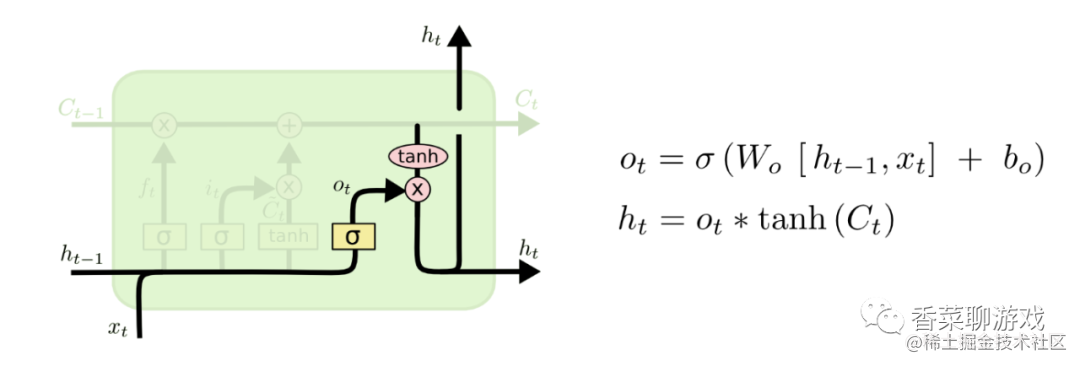Ot是要sigmod神经单元

Ht 是Ct 作为输入的tanh单元

## 4、pytorch 模块 参数

pytorch 提供了 LSTM的实现，所以下面我们说一下参数的解释

``````class torch.nn.LSTM(*args, **kwargs)

input_size：x的特征维度
hidden_size：隐藏层的特征维度
num_layers：lstm隐层的层数，默认为1
bias：False则bihbih=0和bhhbhh=0. 默认为True
batch_first：True则输入输出的数据格式为 (batch, seq, feature)
dropout：除最后一层，每一层的输出都进行dropout，默认为: 0
bidirectional：True则为双向lstm默认为False
input(seq_len, batch, input_size)

batch：每次喂给网络的数据条数，在NLP中就是一次喂给网络多少个句子
input_size：特征维度，和前面定义网络结构的input_size一致。
output,(ht, ct) = net(input)
output: 最后一个状态的隐藏层的神经元输出
ht：最后一个状态的隐含层的状态值
ct：最后一个状态的隐含层的遗忘门值

## 5、lstm 示例

``````import torch
import torch.nn as nn
import torch.nn.functional
import numpy as np
import pandas as pd
import matplotlib.pyplot as plt
"""

"""
dataset = flight_data.values
dataset = dataset.astype('float32')
​
print(flight_data.shape)
​
#绘制每月乘客的出行频率
fig_size = plt.rcParams['figure.figsize']
fig_size = 15
fig_size = 5
plt.rcParams['figure.figsize'] = fig_size
plt.title('Month vs Passenger')
plt.ylabel('Total Passengers')
plt.xlabel('Months')
plt.grid(True)
plt.autoscale(axis='x',tight=True)
plt.plot(flight_data['passengers'])
plt.show()
​
"""

"""
flight_data.columns#显示数据集中 列的数据类型
all_data = flight_data['passengers'].values.astype(float)#将passengers列的数据类型改为float
#划分测试集和训练集
test_data_size = 12
train_data = all_data[:-test_data_size]#除了最后12个数据，其他全取
test_data = all_data[-test_data_size:]#取最后12个数据
print(len(train_data))
print(len(test_data))
​
#最大最小缩放器进行归一化，减小误差
from sklearn.preprocessing import MinMaxScaler
scaler = MinMaxScaler(feature_range=(-1, 1))
train_data_normalized = scaler.fit_transform(train_data.reshape(-1, 1))
#查看归一化之后的前5条数据和后5条数据
print(train_data_normalized[:5])
print(train_data_normalized[-5:])
#将数据集转换为tensor，因为PyTorch模型是使用tensor进行训练的，并将训练数据转换为输入序列和相应的标签
train_data_normalized = torch.FloatTensor(train_data_normalized).view(-1)
#view相当于numpy中的resize,参数代表数组不同维的维度；
#参数为-1表示，这个维的维度由机器自行推断，如果没有-1，那么view中的所有参数就要和tensor中的元素总个数一致
​
#定义create_inout_sequences函数，接收原始输入数据，并返回一个元组列表。
def create_inout_sequences(input_data, tw):
inout_seq = []
L = len(input_data)
for i in range(L-tw):
train_seq = input_data[i:i+tw]
train_label = input_data[i+tw:i+tw+1]#预测time_step之后的第一个数值
inout_seq.append((train_seq, train_label))#inout_seq内的数据不断更新，但是总量只有tw+1个
return inout_seq
train_window = 12#设置训练输入的序列长度为12，类似于time_step = 12
train_inout_seq = create_inout_sequences(train_data_normalized, train_window)
print(train_inout_seq[:5])#产看数据集改造结果
"""

create_inout_sequences返回的元组列表由一个个序列组成，

"""
​
"""

1、input_size:对应的及特征数量，此案例中为1，即passengers
2、output_size:预测变量的个数，及数据标签的个数
2、hidden_layer_size:隐藏层的特征数，也就是隐藏层的神经元个数
"""
class LSTM(nn.Module):#注意Module首字母需要大写
def __init__(self, input_size=1, hidden_layer_size=100, output_size=1):
super().__init__()
self.hidden_layer_size = hidden_layer_size
​
# 创建LSTM层和linear层，LSTM层提取特征，linear层用作最后的预测
##LSTM算法接受三个输入：先前的隐藏状态，先前的单元状态和当前输入。
self.lstm = nn.LSTM(input_size, hidden_layer_size)
self.linear = nn.Linear(hidden_layer_size, output_size)
​
#初始化隐含状态及细胞状态C，hidden_cell变量包含先前的隐藏状态和单元状态
self.hidden_cell = (torch.zeros(1, 1, self.hidden_layer_size),
torch.zeros(1, 1, self.hidden_layer_size))
# 为什么的第二个参数也是1
# 第二个参数代表的应该是batch_size吧
# 是因为之前对数据已经进行过切分了吗？？？？？
​
def forward(self, input_seq):
lstm_out, self.hidden_cell = self.lstm(input_seq.view(len(input_seq), 1, -1), self.hidden_cell)
#lstm的输出是当前时间步的隐藏状态ht和单元状态ct以及输出lstm_out
#按照lstm的格式修改input_seq的形状，作为linear层的输入
predictions = self.linear(lstm_out.view(len(input_seq), -1))
return predictions[-1]#返回predictions的最后一个元素
"""
forward方法：LSTM层的输入与输出：out, (ht,Ct)=lstm(input,(h0,C0)),其中

1、input为（seq_len,batch,input_size）格式的tensor,seq_len即为time_step
2、h0为(num_layers * num_directions, batch, hidden_size)格式的tensor，隐藏状态的初始状态
3、C0为(seq_len, batch, input_size）格式的tensor，细胞初始状态

1、output为(seq_len, batch, num_directions*hidden_size）格式的tensor，包含输出特征h_t(源于LSTM每个t的最后一层)
2、ht为(num_layers * num_directions, batch, hidden_size)格式的tensor，
3、Ct为(num_layers * num_directions, batch, hidden_size)格式的tensor，
"""
​
#创建LSTM()类的对象，定义损失函数和优化器
model = LSTM()
loss_function = nn.MSELoss()
print(model)
​
"""

batch-size是指1次迭代所使用的样本量；
epoch是指把所有训练数据完整的过一遍；

"""
epochs = 300
for i in range(epochs):
for seq, labels in train_inout_seq:
#清除网络先前的梯度值
#初始化隐藏层数据
model.hidden_cell = (torch.zeros(1, 1, model.hidden_layer_size),
torch.zeros(1, 1, model.hidden_layer_size))
#实例化模型
y_pred = model(seq)
#计算损失，反向传播梯度以及更新模型参数
single_loss = loss_function(y_pred, labels)#训练过程中，正向传播生成网络的输出，计算输出和实际值之间的损失值
single_loss.backward()#调用loss.backward()自动生成梯度，
optimizer.step()#使用optimizer.step()执行优化器，把梯度传播回每个网络
​
# 查看模型训练的结果
if i%25 == 1:
print(f'epoch:{i:3} loss:{single_loss.item():10.8f}')
print(f'epoch:{i:3} loss:{single_loss.item():10.10f}')
​
"""

"""
fut_pred = 12
test_inputs = train_data_normalized[-train_window:].tolist()#首先打印出数据列表的最后12个值
print(test_inputs)
​
#更改模型为测试或者验证模式
model.eval()#把training属性设置为false,使模型处于测试或验证状态
for i in range(fut_pred):
seq = torch.FloatTensor(test_inputs[-train_window:])
model.hidden = (torch.zeros(1, 1, model.hidden_layer_size),
torch.zeros(1, 1, model.hidden_layer_size))
test_inputs.append(model(seq).item())
#打印最后的12个预测值
print(test_inputs[fut_pred:])
#由于对训练集数据进行了标准化，因此预测数据也是标准化了的
#需要将归一化的预测值转换为实际的预测值。通过inverse_transform实现
actual_predictions = scaler.inverse_transform(np.array(test_inputs[train_window:]).reshape(-1, 1))
print(actual_predictions)
​
"""

"""
x = np.arange(132, 132+fut_pred, 1)
plt.title('Month vs Passenger  with all data')
plt.ylabel('Total Passengers')
plt.xlabel('Months')
plt.grid(True)
plt.autoscale(axis='x', tight=True)
plt.plot(flight_data['passengers'])
plt.plot(x, actual_predictions)
plt.show()
#绘制最近12个月的实际和预测乘客数量,以更大的尺度观测数据
plt.title('Month vs Passenger  last pred data')
plt.ylabel('Total Passengers')
plt.xlabel('Months')
plt.grid(True)
plt.autoscale(axis='x', tight=True)
plt.plot(flight_data['passengers'][-train_window:])
plt.plot(x, actual_predictions)
plt.show()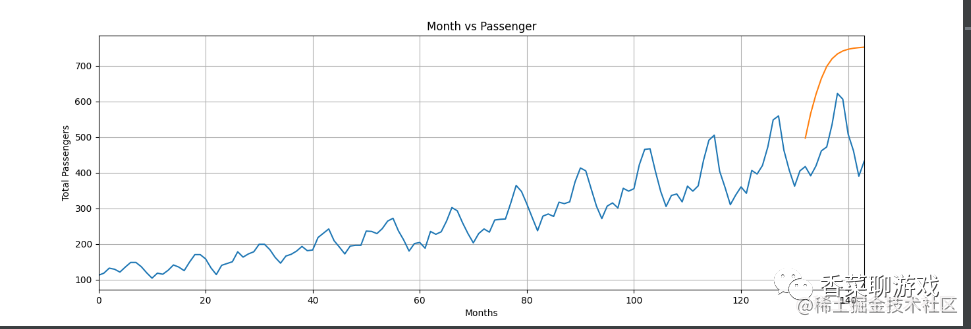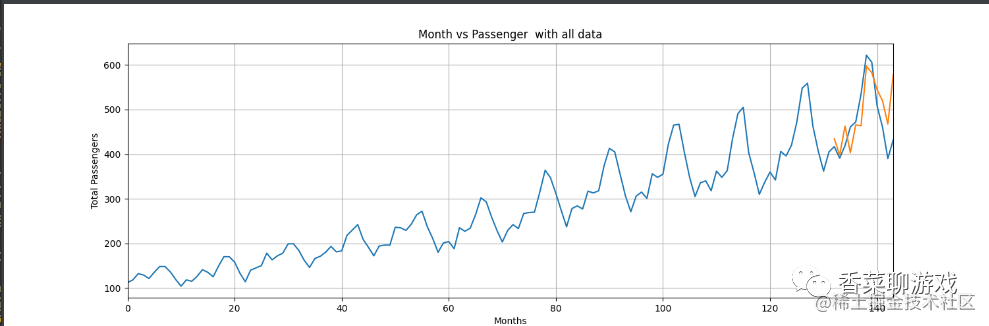源码和数据下载地址：download.csdn.net/download/pe…

## 6、总结

LSTM的三个门是重点，理解了三个门感觉也很简单，但是也因为引入了很多内容，导致参数变多，也使得训练难度加大了很多。因此很多时候我们往往会使用效果和LSTM相当但参数更少的GRU来构建大训练量的模型。下期聊下GRU，一个优化版或者说缩减版的LSTM。